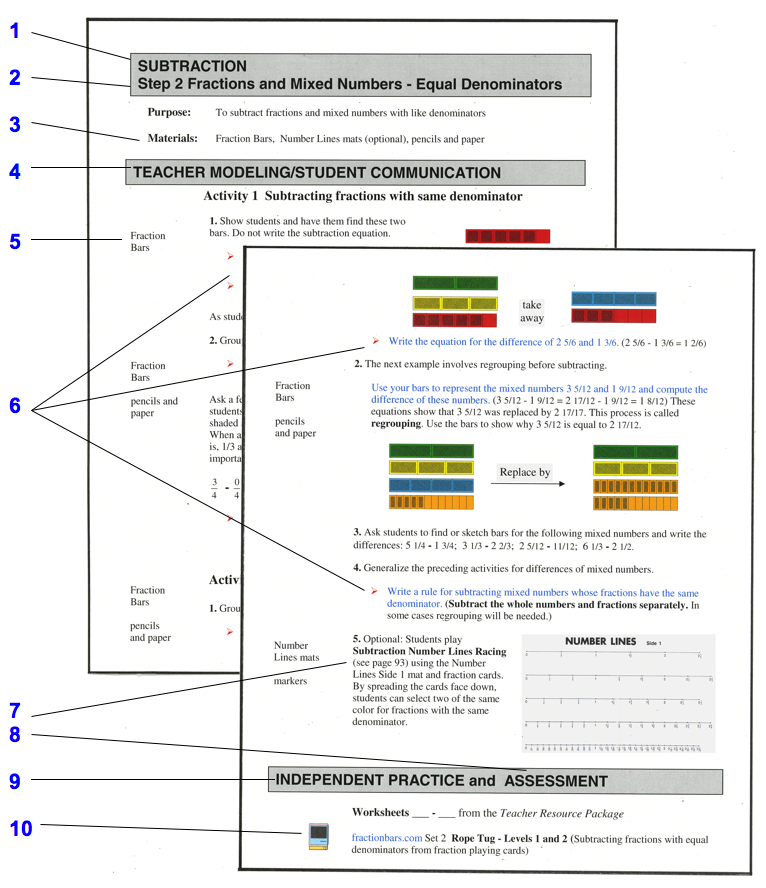Home

Grades 5-8 Fraction Bars Teacher's Guide

Components of Lessons in Fraction Bars Teacher's GuideKEY TO COMPONENTS OF THE LESSONS 1 The teacher's guide is divided into eight main sections:  Basic Concepts; Equality; Inequality; Addition; Subtraction; Multiplication; Division and Fractions - Decimals - Percents. Each step uses concrete models and illustrations before introducing fraction terminology and rules. 2 Each lesson (step) addresses a specific fraction concept and for convenience is contained on two facing pages. Many lessons contain opportunities for students to state generalizations of procedures for comparing fractions and computing with fractions. 3 Materials necessary to teach all the activities in the lesson are listed at the top of each lesson. 4 Teacher Modeling/Student Communication is the part of the lesson that provides basic instruction and activities for the concepts.  Each activity reinforces the Purpose stated at the top of the page.  Students can work in pairs or small groups of three or four to share sets of Fraction Bars, Fraction Cards, or the game/activity mats that are necessary for the lesson. 5 Specific materials necessary for each activity are listed in the left hand columns of the lessons at points of use. Fraction Bars, Fraction Playing Cards, game/activity mats, and markers are some of the materials listed in these columns. There are optional transparencies of the game/activity mats (Transp #1, Transp #2, etc.) and these are also listed in the left hand column as alternative ways of providing demonstrations. The transparent Fraction Bars in this kit are not referenced in the lessons, but in general may be used in place of, or together with, the plastic Fraction Bars for demonstrations. 6 Teacher directions and comments for students are colored blue and marked with red bullets, and information for the teacher is in black font. 7 There are 34 games and one or more are referenced at the end of each lesson. These games are designed to reinforce concepts and provide practice with skills. They also provide opportunities for students to discover and formulate game strategies, many of which involve probability. 8 The last step of each of the eight major sections includes a Performance Test from the Teacher Resource Package for assessing all concepts presented in the section.  There is also an Achievement Test at the end of the Teacher Resource Package for assessing the topics in all eight sections.  This test may be used as a pretest to determine student placement in the program or as a posttest. 9 Activity Sheets for independent practice from the Teacher Resource Package are referenced at the end of each lesson. The Activity Sheets for the last lesson of each of the eight sections involve word problems for all concepts taught in the section. 10 The website fractionbars.com has 16 interactive games that are referenced in appropriate lessons of the first seven sections of the Guide, and decimalsquares.com has 8 interactive games that are referenced in the lessons of the last section of the Guide.